# Miniaturize Patch Antennas Using Metamaterial-Inspired Technique

This example shows how to design a miniaturized patch antenna at 2.4 GHz with a complementary split-ring resonator (CSRR) loading plane and evaluate its performance, with results as published in . The antenna is miniaturized by shrinking its circular patch structure from the original radius of 23.1 mm to 6 mm.

#### Define Patch Dimensions

The microstrip patch in this example has 3 layers: top layer, loading plane, and ground plane. The board is a square with the side length `B_d`. `r_0` is the original radius of the resonant patch in the top layer, which is shrunk to `r`. The CSRR has `N` complementary split rings in the loading plane, with the split width of `w` and the inner and outer radius of `R2_in` and `R2_out`, respectively.

```clc r_0=23.1e-3; r = 6e-3; B_d =2.2*r_0; N=7; w = 0.011*N; R2_in=0.3521; R2_out=(R2_in*N/1.9079)-w;```

#### Design Top Layer

Create a circular patch with the radius of `r`.

`circle_L1= antenna.Circle(Center=[0 0],Radius=r);`

Create the feed as a rectangle.

`rect_L1 = antenna.Rectangle(Length=B_d/2,Width=w*r);`

Move the feed in the x-y plane to connect it to the circular patch.

`translate(rect_L1,[-B_d/4 0 0]);`Create a polygon combining the patch and the feed, and then plot the resulting shape.

```polygon_L1 = circle_L1+rect_L1; show(polygon_L1); title('Top Layer')```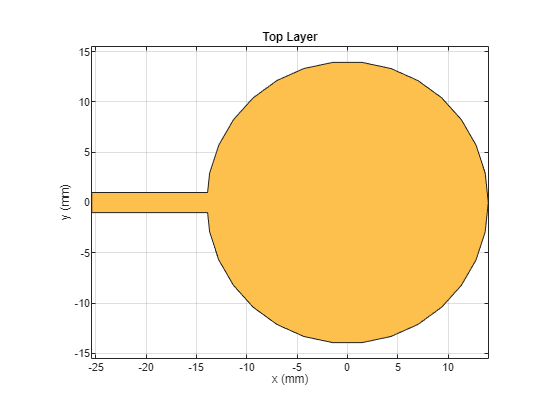To create a CSRR with seven rings, first, create a variable `r_rad` which generates multiplying factors for each of the seven rings. Then use a MATLAB `for` loop to iterativley add and remove circles to create the ring slots that make up the CSRR.

```r_rad=linspace(R2_out,R2_in,N); sign=-1; circ_outer_L2 = antenna.Circle(Center=[0 0],Radius=(R2_out+w)*r); for i=1:length(r_rad) circle_Minus = antenna.Circle(Center=[0 0],Radius=r_rad(i)*r); circle_Plus = antenna.Circle(Center=[0 0],Radius=(r_rad(i)-w)*r); rect_Minus = antenna.Rectangle(Center=[0,sign*(r_rad(i)-w/2)*r],Length=w*r,Width=(w+w/1.3)*r); if i==1 spliti=circ_outer_L2-circle_Minus+circle_Plus+rect_Minus; CSRR_L2=spliti; end CSRR_L2=CSRR_L2-circle_Minus+circle_Plus+rect_Minus; sign=sign*-1; end show(CSRR_L2);title('Loading Plane (CSRR)')```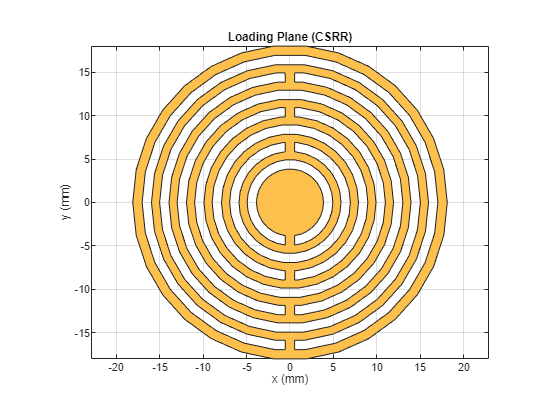#### Design Ground Plane

Design the ground plane as a rectangle with the same dimension as that of the board.

```rect_L3 = antenna.Rectangle(Length=B_d,Width=B_d); show(rect_L3)```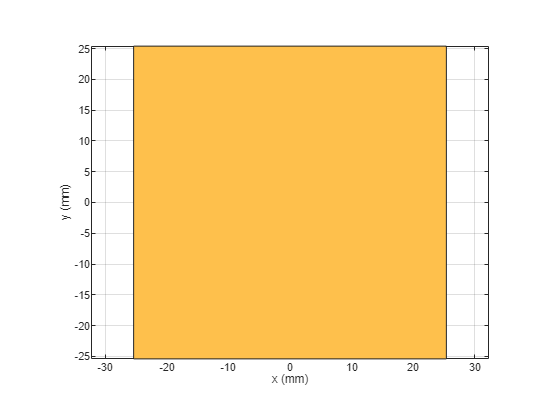#### Design Board

Define the shape of the board and create the stack with the layers mentioned above and specified dielectric layers in between.

`boardShape = antenna.Rectangle(Length=B_d,Width=B_d);`

Create PCB stack using previously defined layers and two dielectric layers.

```p = pcbStack; p.BoardShape = boardShape; d1 = dielectric('FR4'); % d1 = dielectric('RT5870'); d1.Thickness = 2.34e-3; % d2 = dielectric('RT5870'); d2 = dielectric('FR4'); d2.Thickness = 2.34e-3; p.BoardThickness = d1.Thickness+d2.Thickness; p.Layers = {polygon_L1,d1,CSRR_L2,d2,rect_L3}; p.FeedLocations = [-B_d/2 0 1 3]; figure show(p)```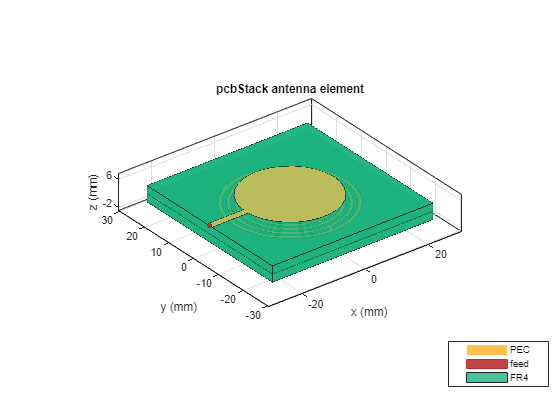#### Analyze Performance of Miniature Antenna

Before evaluating the board's performance, estimate the memory required to solve the mesh structure. You can do this by using the memory estimator.

`memoryEstimate(p,2.4e9)`
```ans = '4.4 GB' ```

Based on the memory estimate, you can utilize parallel computing in MATLAB to accelerate the simulation. For the purpose of this example, load a MAT file containing the return loss computations. You can view the code used to compute the return loss in the Compute Return Loss section.

`load("RL_linear.mat"); `

Plot the return loss of the patch. The patch is resonant at 2.396 GHz, even though its dimension is much smaller than its natural resonant size. Its area is 16 times smaller than the resonant area, as reported in .

```plot(freq,RL_parfor,Marker='o',LineWidth=2); grid on % Create ylabel ylabel({'Magnitude (dB)'},FontWeight='bold',FontSize=14); % Create xlabel xlabel({'Frequency (Hz)'},FontWeight='bold',FontSize=14); % Create title title({'Return Loss'},FontWeight='bold',FontSize=18); % Set XTicks and Yticks set(gca,XTick=decimate(freq,3)) set(gca,YTick=floor(min(RL_parfor))-1:3:ceil(max(RL_parfor)+1))```The linearly spaced frequency points cannot capture the varying resonance between 2.36 GHz and 2.43 GHz. Alternatively, you can use the `densespace` function to generate the 40 frequency points that are dense around the resonant frequency. Call this function instead of the `linspace` in return loss computation. For the purpose of this example, load a MAT file containing the return loss computations done using `densespace` function.

`load("resR23521.mat");`

Plot the return loss with the dense space.

```f = figure; f.Position = [100 100 1820 500]; plot(freq,RL_parfor,Marker='o',LineWidth=2); % Create ylabel ylabel({'Magnitude (dB)'},FontWeight='bold',FontSize=14); % Create xlabel xlabel({'Frequency (Hz)'},FontWeight='bold',FontSize=14); % Create title title({'Return Loss'},FontWeight='bold',FontSize=18); % Set XTicks and Yticks f_points=round(downsample(freq,3),3); % set(gca,'XTick',round(downsample(freq,3),10)) set(gca,XTick=f_points) set(gca,YTick=floor(min(RL_parfor))-1:2:ceil(max(RL_parfor)+1)) grid on```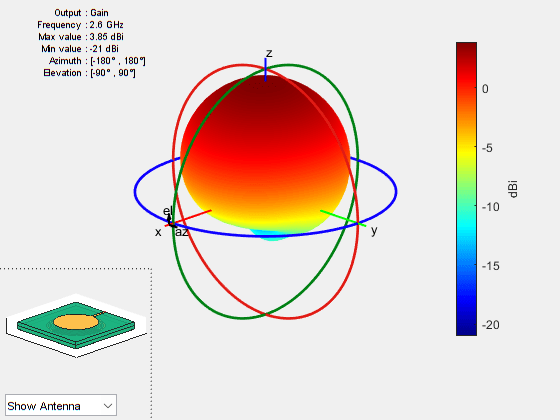Plot the 3-D radiation pattern of the patch.

```f = figure; f.Position = [100 100 720 400]; pattern(p,2.396e9)```Visualize the current density of the patch.

`current(p,2.396e9,scale='log')`#### Compute Return Loss

This example uses the following commented code to compute the return loss of the designed patch antenna.

```%returnLoss(p,linspace(2.25e9,2.55e9,31)); % freq = linspace(2.25e9,2.55e9,40); % RL_parfor = zeros(size(freq)); % % tic; % temp = returnLoss(p, freq(end)); % meshdata = mesh(p); % [~] = mesh(p, 'MaxEdgeLength', meshdata.MaxEdgeLength); % parfor m = 1:numel(freq) % m % RL_parfor(m) = returnLoss(p, freq(m)); % end % time4 = toc```

#### Reference

 Ouedraogo, Raoul O., Edward J. Rothwell, Alejandro R. Diaz, Kazuko Fuchi, and Andrew Temme. “Miniaturization of Patch Antennas Using a Metamaterial-Inspired Technique.” IEEE Transactions on Antennas and Propagation 60, no. 5 (May 2012): 2175–82. https://doi.org/10.1109/TAP.2012.2189699.